General Question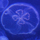How do you work out coefficients?

Asked by Coting (371) February 7th, 2010

E.G.
Find the coefficient of the given X term.

X^3 in the expansion of (1+5)^3

Now I know that answer is 10. but could someone explain how to work out the answer, for future reference?

Observing members: 0Composing members: 0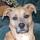First of all, thank you for asking the question this way, so that we can answer without feeling like we’re doing your homework for you. That’s a good thing.

But the downside is… your question doesn’t really make sense. There is no X^3. The expansion of the single term you gave: (1+5)^3 = (6)^3 = 6 * 6 * 6 = 216.

There’s no way I can see to make sense of your statement that “I know that answer is 10”; it just doesn’t compute. There is no “X^3” term in your question. Maybe I’m missing something—it wouldn’t be the first time!—but you have definitely misstated something.

CyanoticWasp (20065)“Great Answer” (4) Flag as…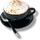@CyanoticWasp, wouldn’t the parens make it 1^3 + 5^3? Making the answer 1+125. x=126? I was wondering how the answer was 10 and if we could get a look at it in its original form….

Trillian (21106)“Great Answer” (0) Flag as…@Trillian nope. The parentheses determine the order of precedence of operations. The operation inside of the parens happens first, and then the raising to the power.

Of course, this could be done as (1+5) * (1+5) * (1+5) and you could cross-multiply if you want (as you have to if the term was, for example, “x+5” instead of “1+5”). But in this case it’s just 6 * 6 * 6.

CyanoticWasp (20065)“Great Answer” (2) Flag as…@CyanoticWasp
Just to say this isn’t homework this is just me wanting to learn.

@Trillian
Also that’s what I thought.

Coting (371)“Great Answer” (0) Flag as…oh the question is wrong it’s (1+x)^5

Coting (371)“Great Answer” (0) Flag as…(1+x)^5

Coting (371)“Great Answer” (0) Flag as…You can see this on Wolfram Alpha. I’m so glad to have that as a reference now.

The cross-multiplication of (1+x)^5 = x^5+5 x^4+10 x^3+10 x^2+5 x+1

You’d have to cross-multiply (1+x) * (1+x) * (1+x) * (1+x) * (1+x), and doing that (I’m not going to!) gives you the result above.

CyanoticWasp (20065)“Great Answer” (1) Flag as…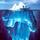This kind of problem ties in with other topics in math, such as Binomial Coefficients and Pascal’s Triangle—there are lots of math sites on the internet geared to various grade levels—just Google around. But it helps to know terminology.

Note the symmetry (1 – 5 – 10 – 10 – 5 – 1) of the coefficients of (x+1)^5. Mathematics is the search for patterns.

gasman (11296)“Great Answer” (1) Flag as…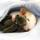(1+X)^3 = C(3,0)x(1^3)x(X^0) + C(3,1)x(1^2)x(X^1) + C(3,2)x(1^1)x(X^2) + C(3,3)x(1^0)x(X^3), where C(n,m) is the binomial coefficient n choose m.

C(n,m) = n!/(m!)x(n-m)!, where n! = nx(n-1)x(n-2)x…x2×1 for n > 0 and 0! = 1.

(1+x)^3 = 1×1x1 + 3×1xX + 3×1x(X^2) + 1×1x(X^3) = 1 + 3xX + 3x(X^2) + (X^3).

That’s the binomial theorem.

ratboy (15157)“Great Answer” (1) Flag as…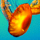@CyanoticWasp : Good job.You saved me some typing.
@gasman and @ratboy : Nice job too.

engineeristerminatorisWOLV (687)“Great Answer” (0) Flag as…@CyanoticWasp. @engineeristerminatorisWOLV: The point of determining the coefficients is to avoid the multiplication by using the binomial theorem.

ratboy (15157)“Great Answer” (0) Flag as…I was also going to say that you could work them out with sweat pants and a professional trainer. I held off this long, but I finally gave in to temptation.

CyanoticWasp (20065)“Great Answer” (0) Flag as…or